Pay

## Order System UML Activity Diagram

32
0
3
publish time: 2020-12-30Captain O Captain

This is an example of UML activity diagram for the process of ordering a product. From this activity diagram, the customer may place order in a complete process.

See More Related TemplatesThis is an example of a UML diagram about adding events to the calendar.
UML Diagram - Add event to calendar
17
1
0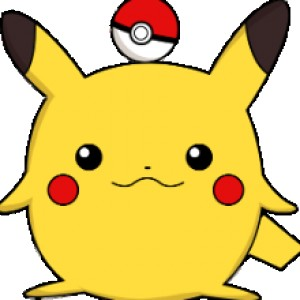easy diagrams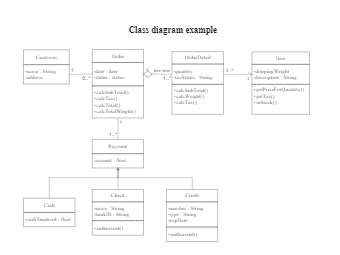This is an example of a uml class diagram.
Class diagram example
165
4
4easy diagrams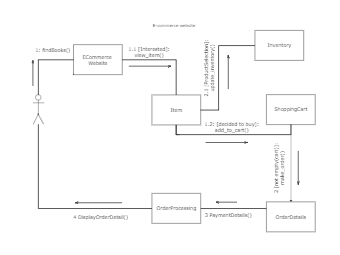This is an example of a UML collaboration diagram.
ecommerce website - UML diagram
10
1
0easy diagrams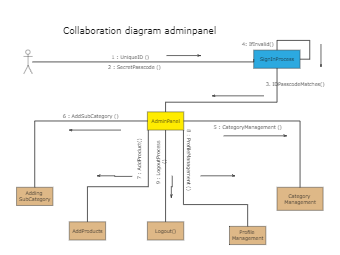6
1
0easy diagrams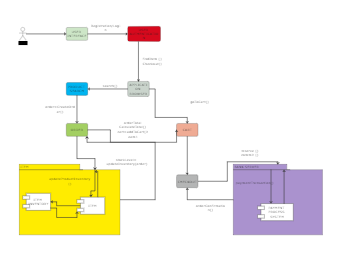This is an example of a UML communication diagram.
Communication diagram example
8
1
0easy diagrams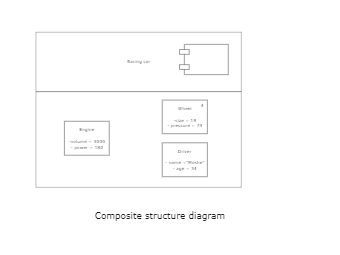This is an example of a composite structure diagram.
Composite structure diagram
3
0
0easy diagrams